Students can download 11th Economics Chapter 6 Distribution Analysis Questions and Answers, Notes, Samcheer Kalvi 11th Economics Guide Pdf helps you to revise the complete Tamilnadu State Board New Syllabus, helps students complete homework assignments and to score high marks in board exams.

## Tamilnadu Samacheer Kalvi 11th Economics Solutions Chapter 6 Distribution Analysis

### Samacheer Kalvi 11th Economics Distribution Analysis Text Book Back Questions and Answers

PART – A

Multiple Choice Questions:

Question 1.
In Economics, distribution of income is among the ……………………….
(a) Factors of production
(b) Individual
(c) Firms
(a) Factors of productionQuestion 2.
Theory of distribution is popularly known as
(a) Theory of product-pricing
(b) Theory of factor-pricing
(c) Theory of wages
(d) Theory of Interest
(b) Theory of factor-pricing

Question 3.
Rent is the reward for the use of ………………………..
(a) Capital
(b) Labour
(c) Land
(d) Organization
(c) LandQuestion 4.
The concept of ‘Quasi-Rent’ is associated with
(a) Ricardo
(b) Keynes
(c) Walker
(d) Marshall
(d) Marshall

Question 5.
The Classical Theory or Rent was propounded by ………………………..
(a) Ricardo
(b) Keynes
(c) Marshall
(d) Walker
(a) RicardoQuestion 6.
‘Original and indestructible powers of the soil’ is the term used by
(a) J.S.Mill
(b) Walker
(c) Clark
(d) Ricardo
(d) Ricardo

Question 7.
The reward for labour is ………………………
(a) Rent
(b) Wage
(c) Profit
(d) Interest
(b) Wage

Question 8.
Money wages are also known as
(a) real wages
(b) nominal wages
(c) original wages
(d) transfer wages
(b) nominal wagesQuestion 9.
Residual Claimant Theory is propounded by ………………………..
(a) Keynes
(b) Walker
(c) Hawley
(d) Knight
(b) Walker

Question 10.
The reward given for the use of capital
(a) rent
(b) wage
(c) interest
(d) profit
(c) interest

Question 11.
Keynesian Theory of interest is popularly known as ………………………
(a) Abstinence Theory
(b) Liquidity Preference Theory
(c) Loanable Funds Theory
(d) Agio Theory
(b) Liquidity Preference TheoryQuestion 12.
According to the Loanable Funds Theory, supply of loanable funds is equal to
(a) S + BC + DH + DI
(b) I + DS + DH + BM
(c) S + DS + BM + DI
(d) S + BM + DH + DS
(a) S + BC + DH + DI

Question 13.
The concept of meeting unexpected expenditure according to Keynes is …………………………
(a) Transaction motive
(b) Precautionary motive
(c) Speculative motive
(d) Personal motive
(b) Precautionary motive

Question 14.
The distribution of income or wealth of a country among the individuals are
(a) functional distribution
(b) personal distribution
(c) goods distribution
(d) services distribution
(b) personal distributionQuestion 15.
Profit is the reward for ………………………..
(a) Land
(b) Organization
(c) Capital
(d) Labour
(b) Organization

Question 16.
Innovation Theory of profit was given by
(a) Hawley
(b) Schumpeter
(c) Keynes
(d) Knight
(b) SchumpeterQuestion 17.
Quasi – rent arises in ………………………..
(c) Imported items
(d) None of these

Question 18.
“Wages as a sum of money are paid under contract by an employer to a worker for services rendered” -Who said this?
(a) Benham
(b) Marshall
(c) Walker
(d) J.S.Mill
(a) Benham

Question 19.
Abstinence Theory of Interest was propounded by …………………………
(a) Alfred Marshall
(b) N.W Senior
(c) Bohm – Bawerk
(d) Knut Wicksell
(b) N.W SeniorQuestion 20.
Loanable Funds Theory of Interest is called as
(a) Classical Theory
(b) Modem Theory
(d) Neo-Classical Theory
(d) Neo-Classical Theory

Part – B

Answer the following questions in one or two sentences.

Question 21.
What is meant by distribution?
Distribution means division of income among the four factors of production as rent, wage, interest and profit.Question 22.
Mention the types of distribution.

1. Personal Distribution
2. Functional Distribution

Question 23.
Define ‘Rent’?
Rent is that part of payment made by a tenant to his landlords for the use of land only.Question 24.
Distinguish between real and money wages?

 Money Wages Real Wages 1. Money wages are referred to the wages paid in terms of money. Real wages are the wages paid in terms of goods and services. 2. Depend upon the standard of living workers in a country. Depend upon the purchasing power of money.

Question 25.
What do you mean by interest?

1. Interest is the reward paid by the borrower to the lender for the use of capital.
2. Interest is the price paid for the use of capital in any market.
3. Generally speaking, interest is a payment made by a borrower to the lender for the money borrowed.Question 26.
What is profit?

1. The entrepreneur coordinates all the other three factors (land, labour and capital) of production.
2. Entrepreneur is rewarded for his services in the form of profit.
3. Profit is a return to the entrepreneur for the use of his entrepreneurial ability.
4. It is the net income of the organizer.
5. Profit is the amount left with the entrepreneur after he has payments made for all the other factors (land, labour and capital) used by him in the production process.

Question 27.
State the meaning of liquidity preference.
Liquidity preference is the preference to have an amount of cash rather than of claims against others.

PART – C

Answer the following questions in a paragraph.

Question 28.
What are the motives of demand for money?
Motives of Demand for Money:
According to Keynes, there are three motives for liquidity preference. They are:

1. The Transaction Motive:

• The transaction motive relates to the desire of the people to hold cash for the current transactions.
• The amount saved under this motive depends on the level of income.
• Mt = f(y)

2. The Precautionary Motive:

• The precautionary motive relates to the desire of the people to hold cash to meet unexpected or unforeseen expenditures such as sickness, accidents, fire and theft. The amount saved for this motive also depends on the level of income.
Mp = f(y)

3. The Speculative Motive:
The speculative motive relates to the desire of the people to hold cash in order to take advantage of market movements regarding the future changes in the price of bonds and securities in the capital market. The amount saved for this motive depends on the rate of interest.
MS = f(i). There is inverse relation between liquidity preference and rate of interest.Question 29.
List the kinds of wages.
1. Nominal wages or money wages :
Nominal wages are referred to as the wages paid in terms of money.

2. Real wages :
Real wages are the wages paid in terms of goods and services. Hence, real wages are the purchasing power of money wages.

3. Piece wages :
Wages that are paid on the basis of quantum of work done.

4. Time wages :
Wages are paid on the basis of the amount of time that the worker works.

Question 30.
Distinguish between rent and quasi – rent?

 Rent Quasi-Rent 1. Rent accrues to land. Quasi-Rent accrues to the man-made appliance. 2. The supply of land is fixed forever. The supply of man-made appliances is fixed for a short period only. 3. It enters into the price. It does not enter into price.Question 31.
Briefly explain the Subsistence Theory of Wages.

• Subsistence theory was first explained by physiocrats and restated by Ricardo.
• According to this theory, wage must be equal to the subsistence level of the labourer and his family.
• If workers are paid higher wages they would be better off and will have large families. Hence, the population would increase, which results in increased supply of labourer and so wages will come down.
• If wages are lower, there would be a reduction in population and thereby the supply of labour falls and wages increase to the subsistence level.
• This theory holds that the wages of workers would not be above or below the subsistence level of the labourer and his family.

Question 32.
State the Dynamic Theory of Profit?
Dynamic Theory of Profit:

• J.B. Clark propounded this theory in 1900. To him, profit is the difference between price and cost of production of the commodity.
• Profit is the reward for dynamic changes in society. He points out that, profit cannot arise in a static society. In static society, everything remains stationary.
• The following changes take place in a dynamic society.
1. The population is increasing.
2. The volume of capital is increasing.
3. Methods of production are improving.
4. Forms of the industrial organization are changing.
5. The wants of consumers are multiplying.Question 33.
Describe briefly the Innovation Theory of Profit.

• Innovation theory was propounded by Joseph. A. Schumpeter.
• Profit is the reward for “Innovation”. Innovation means invention put into commercial practice.
• An innovation may consist of:
1. Introduction of a new product.
2. Introduction of a new method of production.
3. Opening up of a new market.
4. Discovery of new raw materials.
5. Reorganization of an industry/firm.
6. Anyone of these innovations leads to a reduction in the cost of production and thereby brings profit to an entrepreneur.Question 34.
Write a note on the Risk-bearing Theory of Profit?

• Risk – the bearing theory was propounded by F.B.Hawley in 1907.
• Profit is the reward for “risk-taking” in business.
• Every business involves some risks. So risk-taking is an essential function of the entrepreneur and is the basis of profit.
• Higher the risks, the greater are the profit.
• Profit induces entrepreneurs to undertake risks.

PART – D

Answer the following questions on one page.

Question 35.
Explain the Marginal Productivity Theory of Distribution?
Introduction:

1. This theory was developed by Clark, Wicksteed and Walras. The Marginal productivity theory of distribution explains how the prices of various factors of production are determined.
2. This theory explains how rent, wages, interest and profit are determined.
3. This theory is also known as the “General Theory of Distribution ” or “ National Dividend Theory of Distribution”.

Assumptions:

1. All the factors of production are homogenous.
2. Factors of production can be substituted for each other.
3. There is perfect competition both in the factor market and product market.
4. There is perfect mobility of factors of production.
5. There is full employment of factors.
6. This theory is applicable only in the long-run.
7. The entrepreneurs aim at profit maximization.
8. There is no government intervention in fixing the price of a factor.
9. There is no technological change.

Explanation of the Theory:
According to the Marginal Productivity Theory of Distribution, the.price or the reward for any factor of production is equal to the marginal productivity of that factor. Each factor is rewarded according to its marginal productivity.

Marginal Product:
The Marginal Product is also known as “ Marginal Physical Product “ [MPP]. The Marginal Product of a factor of production means the addition made to the total product by the employment of an additional unit of that factor. The Marginal Product may be expressed as MPP, VMP, and MRP.

1. Marginal Physical product [MPP]: The Marginal Physical Product of a factor is the increment in the total product obtained by the employment of an additional unit of that factor.

2. Value of a marginal product [VMP]: The Value of Marginal Product is obtained by multiplying the marginal physical product of the factor by the price of the product. Symbolically VMP = MPP × Price

3. Marginal Revenue product [MRP]: The Marginal Revenue Product of a factor is the increment in the total revenue which is obtained by the employment of an additional unit of that factor.
MRP = MPP × MR

The Marginal Productivity Theory of Distribution states that

1. The price of a factor of production depends upon its productivity.
2. The price of a factor is determined by and will be equal to the marginal revenue product of that factor.
3. Under certain conditions, the price of a factor will be equal to both the average and marginal products of that factor.Question 36.
Illustrate the Ricardian Theory of Rent?

1. The Classical Theory of Rent is called the “Ricardian Theory of Rent.”
2. “ Rent is that portion of the produce of the earth which is paid to the landlord for the use of the original and indestructible powers of the soil” – David Ricardo

Assumptions:
Ricardian theory of rent assumes the following:

1. Land differs in fertility.
2. The law of diminishing returns operates in agriculture.
3. Rent depends upon fertility and location of the land.
4. The theory assumes perfect competition.
5. It is based on the assumption of a long period.
6. There is the existence of marginal land or no-rent land.
7. The land has certain “Original and indestructible powers”.
8. The land is used for cultivation only.
9. Most fertile lands are cultivated first.

Statement of the Theory with Illustration:
There are three grades of land, namely A, B, and C on that island. ‘A’ being most fertile ‘B’ less fertile and ‘C’ the least fertile. They will first cultivate all the most fertile land. The yield per acre on ‘A’ grade land is 40 bags of paddy.

The same amount labour and capital employed in ‘A’ grade land. The yield per acre on ‘B’ grade land is 30 bags of paddy.
The Surplus of 10 bags [40-30] per acre appears on ‘A’ grade land. This is “ Economic Rent” land of ‘A’ grade land.

The yield per acre on ‘C’ grade land is 20 bags of paddy. This surplus of ‘A’ grade land is now raised to 20 bags [40-20] and it is the “ Economic Rent” of ‘A’ grade land.

The ‘C’ grade land, cost of production is just equal to the price of its products and therefore does not yield any rent [20-20], Hence, ‘C’ grade land is called ‘no-rent land or marginal land ’. The land which yields rent is called “intra-marginal land”.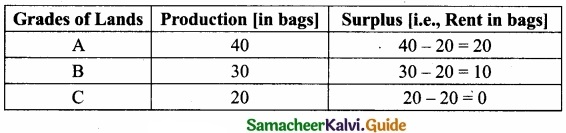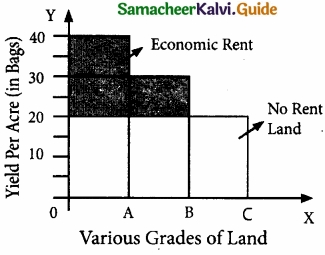Diagrammatic Explanation:

1. In the diagram, X-axis represents various grades of land, and Y-axis represents yield per acre [in bags],
3. The ‘C’ grade land is the no rent land.”
4. ‘A’ land ‘B’ grade lands are “intra – marginal lands”.
5. The economic rent yielded by ‘A’ and ‘B’ grade lands is equal to the shaded area of their respective rectangles.Question 37.
Elucidate the Loanable Funds Theory of Interest?

1. The loanable fund’s theory, also known as the “Neoclassical theory”. This theory was developed by Swedish economists like Wicksell, Bertilohlin, Viner, Gunnar Myrdal, and others.
2. Interest is the price paid for the use of loanable funds.
3. The rate of interest is determined by the equilibrium between the demand for and supply of loanable funds in the credit market.

Demand for loanable funds :

1. Demand for Investment (I)
2. Demand for Consumption (C)
3. Demand for Hoarding (H)

Supply of loanable funds :
1. Savings (S) :
Savings may be of two types, namely.

1. Savings planned by individuals are “ex-ante savings”. (Eg.) LIC premium
2. Unplanned savings are called “ex-post savings”

2. Bank credit:
Commercial banks create credit and supply loanable funds to the investors.

3. Dishoarding (DH) :
Dishoarding means bringing out the hoarded money into use and thus it constitutes a source of supply of loanable funds.

4. Disinvestment (DI):
Disinvestment is the opposite of investment. It means not providing sufficient funds for the depreciation of equipment.

Explanation:
The X-axis represents the demand for and supply of loanable funds, Y-axis represents the rate of interest. The LS curve represents the total supply curve of loanable funds. The LD curve represents the total demand for loanable funds. The LD and LS curves, intersect each other at the point “E” the equilibrium point. At this point OR rate of interest and OM is the number of loanable funds.

Criticisms :

1. Many factors have been included in this theory’. Still, there are many more factors like
• Asymmetric information
• Moral Hazard.
2. It is very difficult to combine real factors with monetary factors.Question 38.
Explain the Keynesian Theory of Interest?
Keynes’ Liquidity Preference Theory of Interest or The monetary Theory of Interest

1. Keynes propounded the Liquidity Preference Theory of Interest in his famous book,
“ The General Theory of Employment, Interest, and Money “ in 1936.
2. According to Keynes, interest is purely a monetary phenomenon because the rate of interest is calculated in terms of money.
3. “Interest is the reward for parting with liquidity for a specified period of time”.

Meaning of Liquidity Preference:

1. Liquidity preference means the preference of the people to hold wealth in the form of liquid cash rather than in other non-liquid assets like bonds, securities, bills of exchange, land, building, gold etc.
2. “Liquidity Preference is the preference to have an amount of cash rather than of claims against others”. – Meyer.

Motives of Demand for Money:
According to Keynes, there are three motives for liquidity preferences. They are:

1. The Transaction Motive:
The transaction motive relates to the desire of the people to hold cash for the current transactions [or-day-to-day expenses] M = f(y)

2. The Precautionary Motive:

• The precautionary motive relates to the desire of the people to hold cash to meet unexpected or unforeseen expenditures such as sickness, accidents, fire, and theft.
• The amount saved for this motive also depends on the level of Income Mp = f(y).

3. The Speculative Motive:

• The speculative motive relates to the desire of the people to hold cash in order to take advantage of market movements regarding the future changes in the price of bonds and securities in the capital market. M = f(i)
• There is an inverse relation between liquidity preference and rate of interest.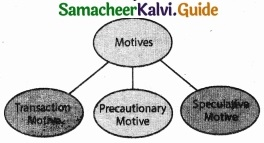### Samacheer Kalvi 11th Economics Distribution Analysis Additional Important Questions and Answers

Part – A

Multiple Choice Questions:

Question 1.
The theory of factor prices is popularly known as the theory of _______
(a) Distribution
(b) Exchange
(c) Wages
(d) Profit
(a) DistributionQuestion 2.
Net profit is otherwise called ………………………
(a) Profit
(b) Risk profit
(c) Dynamic profit
(d) Pure profit
(d) Pure profit

Question 3.
F.A. Walker wrote a book _______ in 1875.
(a) Political economy
(b) Social economy
(c) Principles of economics
(d) Wealth of nations
(a) Political economy

Question 4.
Which is the gift of nature?
(a) Land
(b) Interest
(c) Profit
(d) Capital
(a) LandQuestion 5.
Keynes liquidity preference theory is also called as_______
(a) Classical theory of interest
(b) Psychological theory of interest
(c) The monetary theory of interest
(d) Abstinence theory of interest
(c) The monetary theory of interest

Question 6.
Which one is considered a homogeneous factor?
(a) Labour
(b) Land
(c) Capital
(d) All the above
(d) All the above

Question 7.
Risk bearing theory of profit was propounded by _______
(a) J.B. Clark
(b) J.M. Keynes
(c) F.B. Hawley
(d) H.Knight
(c) F.B. HawleyQuestion 8.
What is the payment for the service of labour?
(a) Wages
(b) Income
(c) Salary
(d) Profit
(a) Wages

Question 9.
_______ is the produced means of production.
(a) Land
(b) Labour
(c) Capital
(d) Organisation
(c) CapitalQuestion 10.
Organization is done by the ………………………
(a) Private sector
(b) Public sector
(c) Service sector
(d) Entrepreneur
(d) Entrepreneur

PART – B

Answer the following questions in one or two sentences.

Question 1.
Define Marginal product?

1. The marginal product of a factor of production means the addition made to the total product by the employment of an additional unit of that factor.
2. The Marginal Product may be expressed as MPP, VMP, and MRP.

Question 2.
What are the other names of the marginal productivity theory of distribution?

1. The general theory of distribution
2. National dividend theory of distributionQuestion 3.
What do you mean by the Marginal Productivity theory of distribution?

1. Marginal Productivity Theory of Distribution was developed by Clark, Wicksteed, and Walras.
2. This theory explains how the prices of various factors of production are determined.
3. This theory explains how rent, wages, interest, and profit are determined.
4. This theory is also known as the “General Theory of Distribution” or “National Dividend Theory of Distribution”.

PART – C

Answer the following questions in a paragraph.

Question 1.
List out the Concepts of Profit?
Concepts of Profit:

1. Gross Profit:
Gross Profit is the surplus that accrues to a firm when it subtracts its Total expenditure from its Total Revenue.
Gross Profit = Total Revenue – Total cost

2. Net profit or Pure Profit or Economic Profit or True Profit:
Net or pure or economic or true profit is the residual left with the entrepreneur after deducting from Gross profit The remuneration for the self-owned factors of production which are called implicit cost.
Net Profit = Gross Profit – implicit costs.

3. Normal Profit:

4. SuperNormal Profit:
Supernormal profits are over and above the normal profit.
SuperNormal Profit = Actual profit – Normal profitQuestion 2.
Describe briefly the equilibrium between Demand and Supply of Money?
1. The equilibrium between liquidity preference and demand for money determines the rate of interest.
2. In the short – run, the supply of money is assumed to be constant.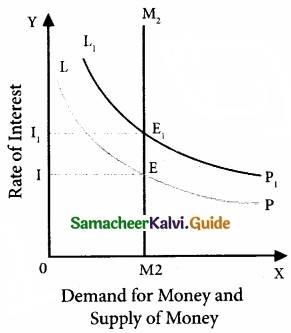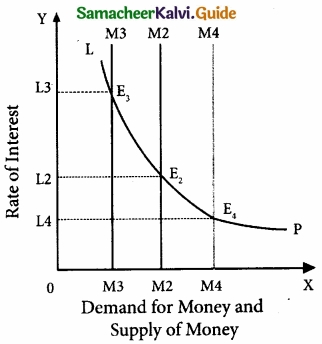3. LP is the liquidity preference curve [demand curve.]

4. M2M2 shows the supply curve of money to satisfy speculative motives. Both curves intersect at point E, which is the equilibrium point. Hence, the rate of interest is 2.5. If liquidity preference increases from LP to L1P1 the supply of money remains constant, the rate of interest would increase from OI to OI1.

5. Suppose LP remains constant. If the supply of money is OM2, the interest is OI2 and if the supply of money is reduced from OM2 to OM4, the interest would increase from OI2 to OI3. If the supply of money is increased from OM2 to OM4, the interest could decrease from OI2 to OI4.Question 3.
Define the kinds of interest.
Gross interest:
Gross interest is the total interest amount received by creditors from debtors.
Gross interest = Net interest + reward for inconvenience + insurance against the risk of non-repayment + payment for service of debt management.

Net interest:
Net interest is only a part of the gross interest. It is the payment for use of capital only. (Eg.) Interest payable for government securities.

PART – D

Answer the following questions on one page.

Question 1.
Explain the classical theory of Interest?
The classical theory of Interest:

1. The equilibrium interest rate, according to classical theory, is determined by the intersection of demand and supply curves, Demand for money refers to investment.
2. The supply of money is referred to as savings. S = I.

Equilibrium:

1. The rate of interest is determined by the equilibrium between the total demand for and the total supply of loanable funds.
2. Supply of and Demand for Loanable funds:
3. Supply of loanable funds = Savings + Bank Credit + Dishoarding + Disinvestment = S + BC + DH + DI
4. Demand for loanable funds = Investment + consumption + Hoarding = I + C + H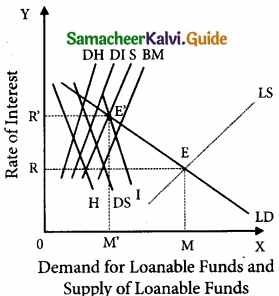• In Diagram X-axis represents the demand for and supply of loanable funds and the Y-axis represents the rate of interest.
• The LS curve represents the total supply curve of loanable funds.
• The summation of the Saving Curve [S], Bank credit curve [BC], Dishoarding curve [DH], and Disinvestment curve [DI],
• The LD curve represents the total demand for loanable funds.
• This is obtained by the summation of the demand for investment curve I, the demand curve for consumption demand or dissaving curve, and the curve for the demand for hoarding curve H.
• The LD and LS curves, intersect each other at the point “E” the equilibrium point.
• At this point, the OR rate of interest and OM is the number of loanable funds.Question 2.
Illustrate the uncertainty Bearing Theory of profit?
Uncertainty theory was propounded by the American economist Frank H.Knight. Profit is the reward for “ uncertainty bearing”. He distinguishes between “insurable” and “non-insurable” risks.

Insurable Risks:

1. Certain risks are measurable or calculable.
2. Some of the examples of these risks are the risk of fire, theft, and natural disasters.
3. Such risks are compensated by the Insurance companies.

Non-Insurable Risks:

1. There are some risks which are immeasurable or incalculable.
2. Examples of these risks are competition,, market condition, technology change, and public policy.
3. No Insurance Company can undertake these risks.
4. The term “risks” covers the first type of events (measurables-insurable)
5. The term “uncertainty” covers the second type of events (unforeseeable or incalculable or not measurable or non-insurable).
6. According to Knight, profit does not arise on account of risk-taking, because the entrepreneur can guard himself against risk by taking a suitable insurance policy.
7. Uncertain events cannot be guarded against in that way.
8. An entrepreneur takes himself the burden of facing an uncertain event, he secures remuneration.
9. That remuneration is “profit”.